# Introduction

## Algebraic equations with solved questions

Algebra is branch of Mathematics in which we should study more as a subject for mental discipline than for anything else. Algebra is a langue to understand mathematics or convert our thought to mathematical expression. Algebra, like arithmetic is a science of numbers with the distinction that the numbers in algebra are generally denoted by letters instead of by figures .We have to understand basic terminology before starting this exercises.

Symbols: The letters of the alphabet a, b, c, ….. are used to denote numbers and the signs

+, –, ×, ÷, =, ….. and are used either to denote operations to be performed upon the number to which they are attached or as abbreviations. These letters and signs are called symbols.

Like: 5a, ab + c × d, 8cf/g.

In the given example 5a means letter a is multiplied by 5.

In the second example ab + c × d ,two form of multiplication are given in the first ab denotes quantity a and b are multiply together but in second case c × d meaning is same as first one i.e. quantity c and d are multiply together in different form . overall a and b multiply together and c and d multiply together and sum of both is represented as ab + c × d or a×b + cd or a×b + c × d , ab + cd.

An equation is a complete mathematical sentence that includes a sign of equality (like an equal sign).

Like: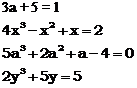An expression is a mathematical sentence; it doesn’t have an equal sign.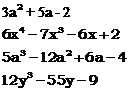Now we know that any intelligible collection of letters, numerals and signs of operations is called an Algebraically expression. Such collection is also sometimes called an Algebraically quantity, or briefly, quantity.

The parts of an algebraically expression that are connected by the signs + are – are called its terms.

Ex : 5a + ab + c × d – 8c × f × g is an algebraically expression of which terms are 5a, ab + c × d, 8c × f ÷ g is an algebraically expression of which terms of 5a, ab + c × d, 8c × f ÷ g.

### Different terminology are used for Expression and Term like:

There are three parts of most expressions: the variable, constant, and coefficient.

Variable: An unknown or changing number. Often represented by “x”.

Constant: A number that doesn’t change

Coefficient: The number that is multiplied by the variable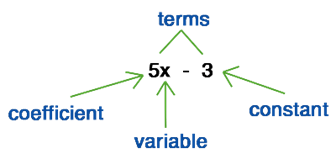Quantity: Anything that can be represented by a number is called a quantity

Ex : Time, weight, money, distance etc. Quantity is also often used in the sense of number, integral or fractional. An algebraic expression also is some times called a quantity.

Variables : Any expression involving a letter is called a function of that letter. Thus,

x3 + 5x + 8 is a function of x ; a2 + ab + b2 is a function of a and b ; a3 + b3 + c3 + 2abc is a function of a, b and c and so on.

The letters of which a function consists are called its variables.

Thus, x2 + 5xy + y2 is a function of which the variables are x and y.

Expressions are either simple or compound. A simple expression is one which has no parts no parts connected by the sign + or –, i.e., which consists of only one term, as monomial.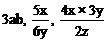and is also called a

A compound expression consists of two or more terms, if it consist of two terms, as 2a + 5bcd,it is called a binomial.

f of three terms, as a + bc + 8efg, x × y ÷ z + a × c –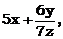, a Trinomial.

And if of more than three terms, a Multinomial or a polynomial.Let’s break it down further.

An expression is made up of terms.

Terms are each separate values in an expression. They can be separated by operations, like plus and minus signs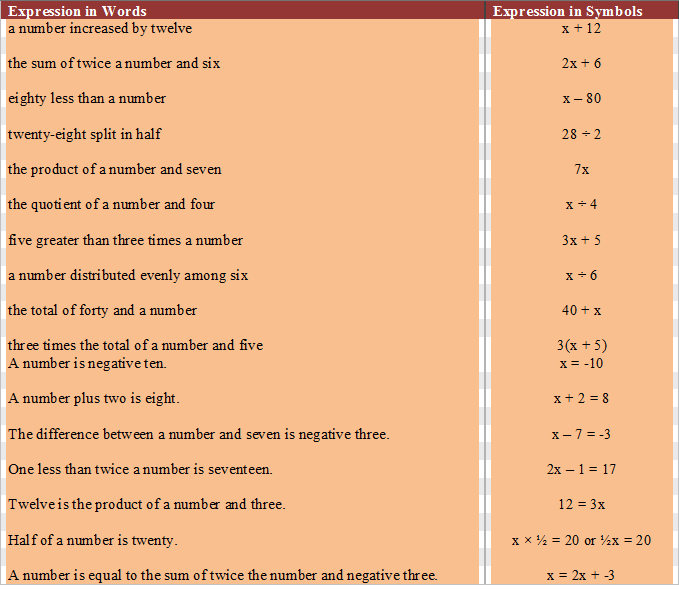To score More in your class 7 refer NCERT solutions for class 7 Maths